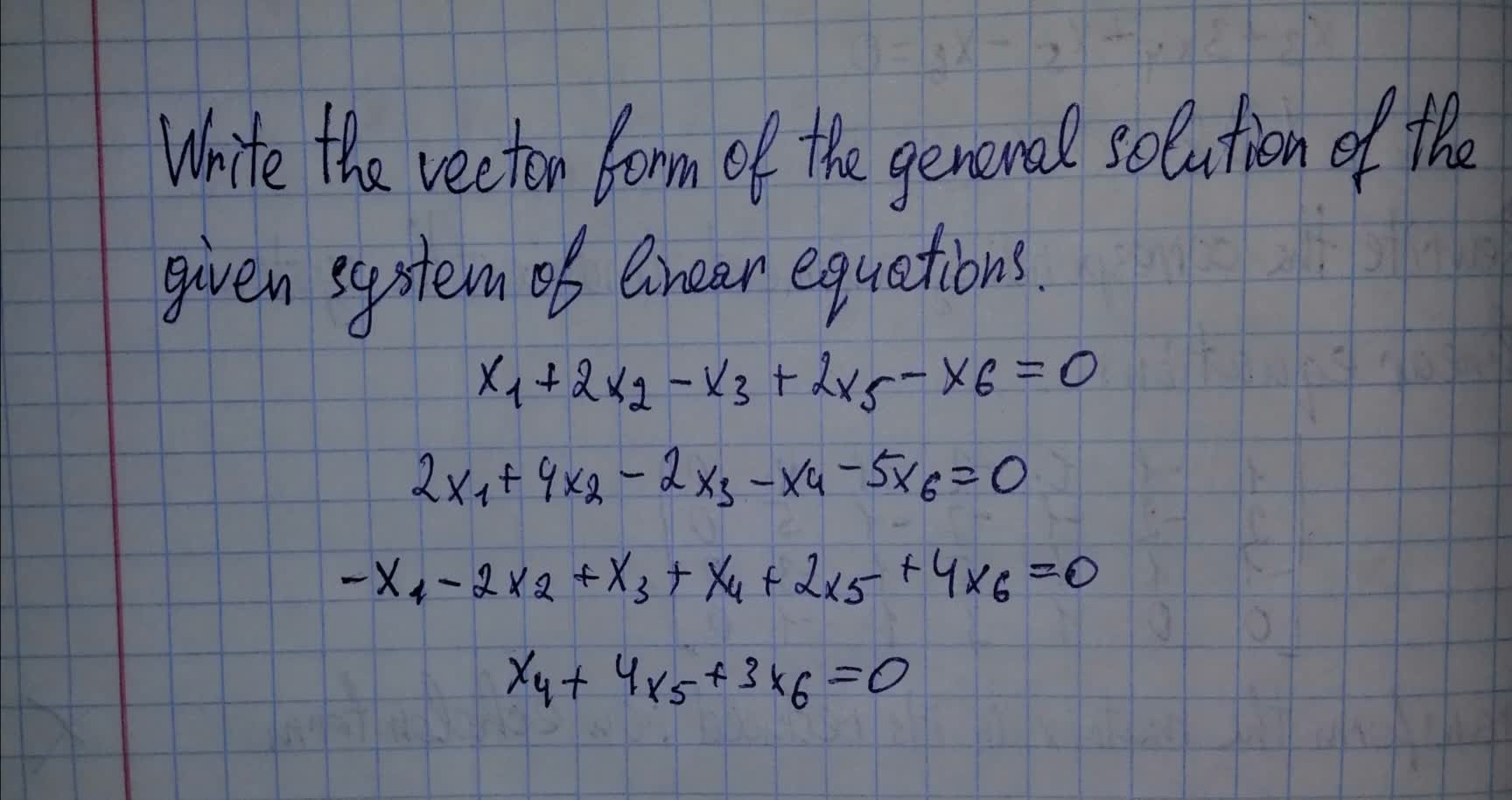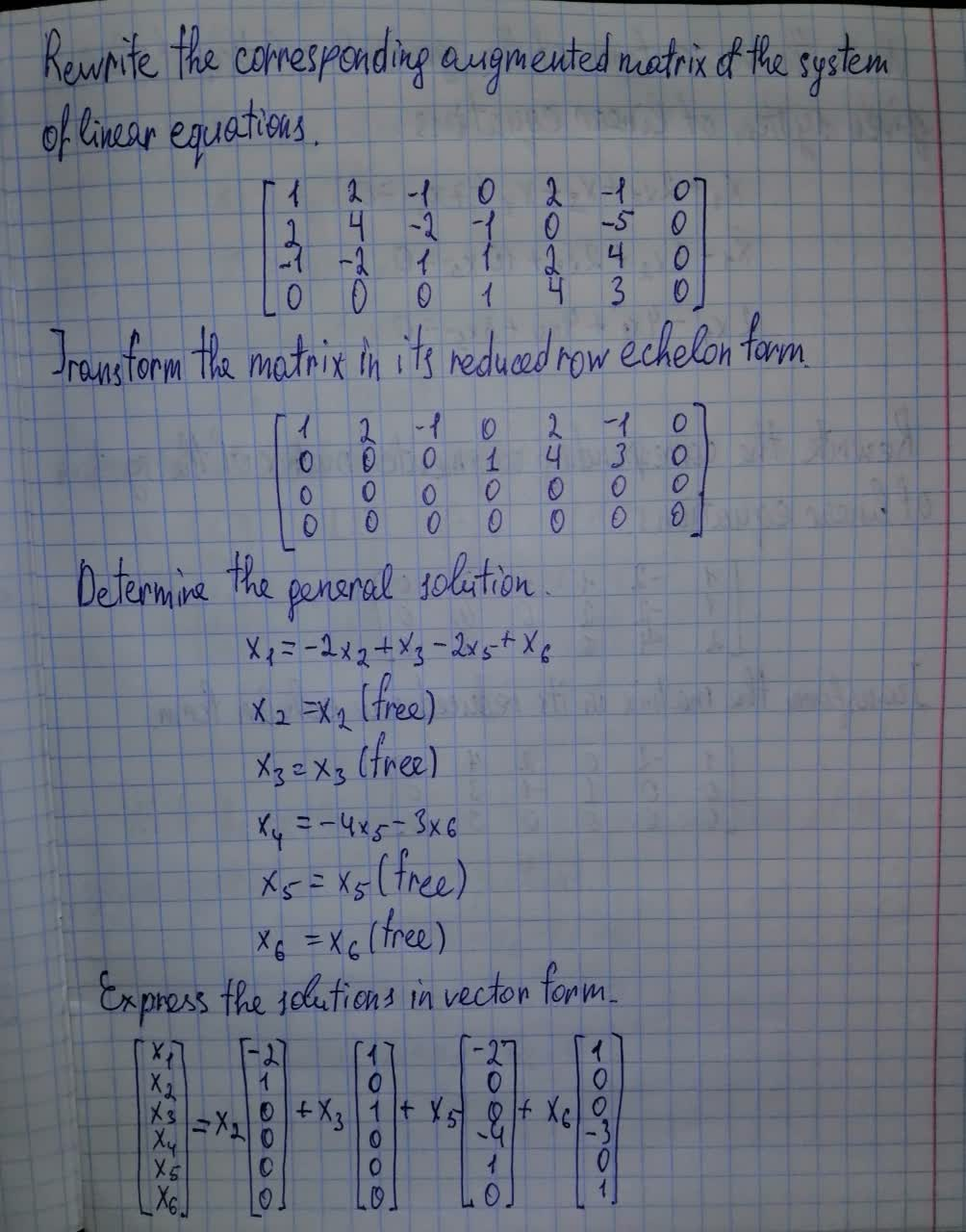Question# Write the vector form of the general solution of the given system of linear equations. x_1+2x_2-x_3+2x_5-x_6=0

Forms of linear equations
ANSWEREDWrite the vector form of the general solution of the given system of linear equations. $$\displaystyle{x}_{{1}}+{2}{x}_{{2}}-{x}_{{3}}+{2}{x}_{{5}}-{x}_{{6}}={0}$$